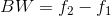# Calculate 3dB Bandwidth and Cutoff Frequency

A cutoff frequency is a border in a system's frequency response at which energy flowing through the system starts to decrease or reflected without being transmitted.
Formula:Where, BW=Bandwidth of a circuit in unit of frequency, fo=center frequency, f2=the upper cut off frequency, f1=the lower cut off frequency

 Enter Center Frequency (f0) = Hz Enter Quality Factor (Q) = Lower cut off frequency (f1) = Hz Upper cut off frequency (f2) = Hz 3 dB Bandwidth (BW) = Hz